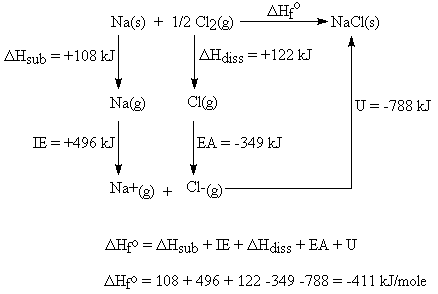QuestionAnswers

# What is lattice enthalpy? How do you calculate the lattice enthalpy of sodium chloride by using Born-Haber’s cycle?Hint: Lattice enthalpy is related to the formation of crystalline solid from its ions. The Born-Haber cycle is a stepwise process to calculate lattice enthalpy. Now, try to answer this question accordingly.

Let’s talk about the Lattice Enthalpy -

Lattice Enthalpy - The lattice enthalpy of a crystalline solid is a measure of the energy released when the ions are combined to make this compound.

The lattice enthalpy is indirectly determined by the use of the Born-Haber Cycle. This procedure is based on Hess's law.

Now, Let’s find the lattice enthalpy of sodium chloride by using Born-Haber’s cycle -
We begin by writing the formation reaction, which is by definition from the elemental states at 25$^{ 0 }$C and 1 atm:

Our goal is to transform the reactants into their ionic gases, as that is the reaction that describes the process for which "lattice energy" is defined.

* Solid sodium atom sublimes to gaseous atom by absorbing heat energy.
$Na(s)\quad \rightarrow\quad Na(g),\quad \Delta H_{ sub,Na(s) }\quad =\quad +108kJ$
* Gaseous sodium atoms absorb the ionization energy to release one electron and form gaseous sodium ions.
$Na(g)\quad \rightarrow\quad Na^{ + }(g)\quad +\quad e^{ - },\quad IE_{ 1,Na(g) }=\quad +496kJ$
* Diatomic gaseous chlorine breaks into two individual atoms by absorbing bond energy, such that each chlorine atom absorbs half of the bond energy of chlorine molecules.
$\dfrac { 1 }{ 2 } Cl_{ 2 }(g)\quad \rightarrow\quad Cl(g),\quad \dfrac { 1 }{ 2 } \Delta H_{ dissociation,Cl_{ 2 }(g) }=\dfrac { 1 }{ 2 } \times 244kJ\quad =\quad +122kJ$
* Chlorine atoms accept an electron to form chloride ions and release energy equivalent to electron affinity.
$Cl(g)\quad +\quad e^{ - }\quad \rightarrow\quad Cl^{ - }(g),\quad EA_{ 1,Cl(g) }\quad =\quad -349kJ$
* Gaseous sodium ion and gaseous chloride ion combine to form a solid sodium-chloride molecule and release energy equivalent to lattice energy.
$Na^{ + }(g)\quad +\quad Cl^{ - }(g)\quad \rightarrow \quad NaCl(s),\quad \Delta H_{ Lattice }\quad =\quad ?$
Summation of enthalpy of all the processes from all five steps gives the net enthalpy of formation of solid crystalline sodium chloride from sodium and chlorine in their standard conditions of solid and gas respectively.
For 1 mol of NaCl(s) -

$Na(s)\quad +\quad \dfrac { 1 }{ 2 } Cl_{ 2 }(g)\quad \rightarrow\quad NaCl(s),\quad \Delta Hf_{ ,NaCl(s) }=\quad -411kJ$
The enthalpies are represented as a cycle in the figure.So, $\Delta H_{ f,NaCl(s) }\quad =\quad \Delta H_{ sub,Na(s) }+IE_{ 1,Na(g) }+\dfrac { 1 }{ 2 } \Delta H_{ dissociation,Cl_{ 2 }(g) }+EA_{ 1,Cl(g) }+U$
or, $\Delta H_{ f,NaCl(s) }-(\Delta H_{ sub,Na(s) }+IE_{ 1,Na(g) }+\dfrac { 1 }{ 2 } \Delta H_{ dissociation,Cl_{ 2 }(g) }+EA_{ 1,Cl(g) }+U)\quad =\quad 0$
or, Lattice enthalpy (U or $\Delta H_{ Lattice }$ ) of the sodium chloride solid ,
$U\quad =\quad \Delta H_{ f,NaCl(s) }-(\Delta H_{ sub,Na(s) }+IE_{ 1,Na(g) }+\dfrac { 1 }{ 2 } \Delta H_{ dissociation,Cl_{ 2 }(g) }+EA_{ 1,Cl(g) })$
U = -411 - (108+496+122-349)
U = -411 -108 -496 -122 +349
U = – 788 kJ/mol
Therefore, we defined the lattice enthalpy and calculated its value for Sodium Chloride.

Note: Hess law’s statement, “The enthalpy change of a reaction is the same at constant volume and pressure whether it takes place in single or multiple steps long as the initial reactants and the final products remain the same.”
Lattice energy is relevant to many practical properties including solubility, hardness, and volatility.

View Notes
How Do Humans Use Microbes?How Do Plants Reproduce - Short AnswerIonization Enthalpy and ValencyWater Cycle ProcessWhat is the Cell Envelope?What is the Placebo EffectWhat is the full form of phd?Why do we Have Two Eyes?Do All Bacteria Have PlasmidsNCERT Book Class 11 Chemistry PDFImportant Questions for CBSE Class 11 Chemistry Chapter 11 - The p-Block ElementsImportant Questions for CBSE Class 10 Science Chapter 8 - How do Organisms Reproduce?Important Questions for CBSE Class 11 ChemistryImportant Questions for CBSE Class 11 Chemistry Chapter 10 - The s-Block ElementsImportant Questions for CBSE Class 11 Chemistry Chapter 14 - Environmental ChemistryImportant Questions for CBSE Class 11 Chemistry Chapter 13 - HydrocarbonsImportant Questions for CBSE Class 11 Chemistry Chapter 1 - Some Basic Concepts of ChemistryImportant Questions for CBSE Class 11 Chemistry Chapter 6 - ThermodynamicsImportant Questions for CBSE Class 11 Chemistry Chapter 7 - EquilibriumImportant Questions for CBSE Class 11 Chemistry Chapter 9 - HydrogenChemistry Question Paper for CBSE Class 12CBSE Class 12 Chemistry Question Paper 2020Chemistry Question Paper for CBSE Class 12 - 2013Chemistry Question Paper for CBSE Class 12 - 2015CBSE Class 12 Chemistry Question Paper 2019 - Free PDFCBSE Class 12 Chemistry Question Paper 2017 - Free PDFCBSE Class 12 Chemistry Question Paper 2018 - Free PDFPrevious Year Question Paper for CBSE Class 12 Chemistry - 2014Chemistry Question Paper for CBSE Class 12 - 2016 Set 1 EChemistry Question Paper for CBSE Class 12 - 2016 Set 1 SNCERT Solutions for Class 10 Science Chapter 8Lakhmir Singh Biology Class 10 Solutions Chapter 3 - How do Organisms Reproduce?NCERT Solutions for Class 11 Chemistry Chapter 11 The p-Block Elements In HindiNCERT Solutions Class 11 ChemistryNCERT Solutions for Class 9 English Beehive Chapter 11 - If I Were YouNCERT Solutions for Class 11 Chemistry Chapter 11NCERT Exemplar for Class 10 Science Chapter 8 - How do Organisms Reproduce? (Book Solutions)NCERT Solutions for Class 10 Science Chapter 8 How do Organisms Reproduce? in HindiEquilibrium NCERT Solutions - Class 11 ChemistryNCERT Exemplar for Class 11 Chemistry Chapter-11 (Book Solutions)